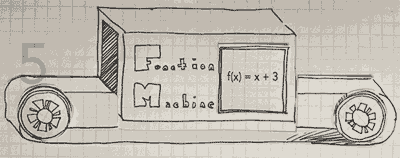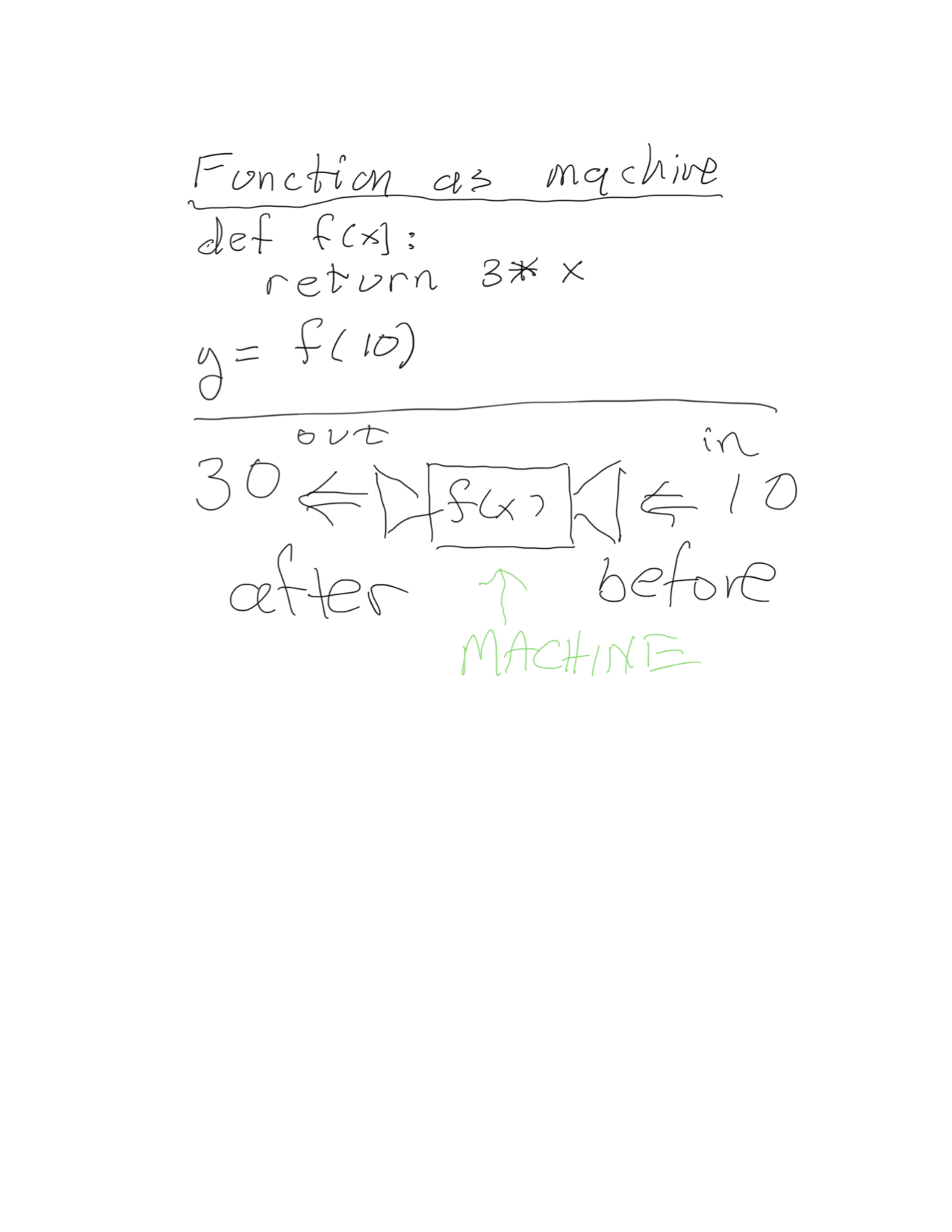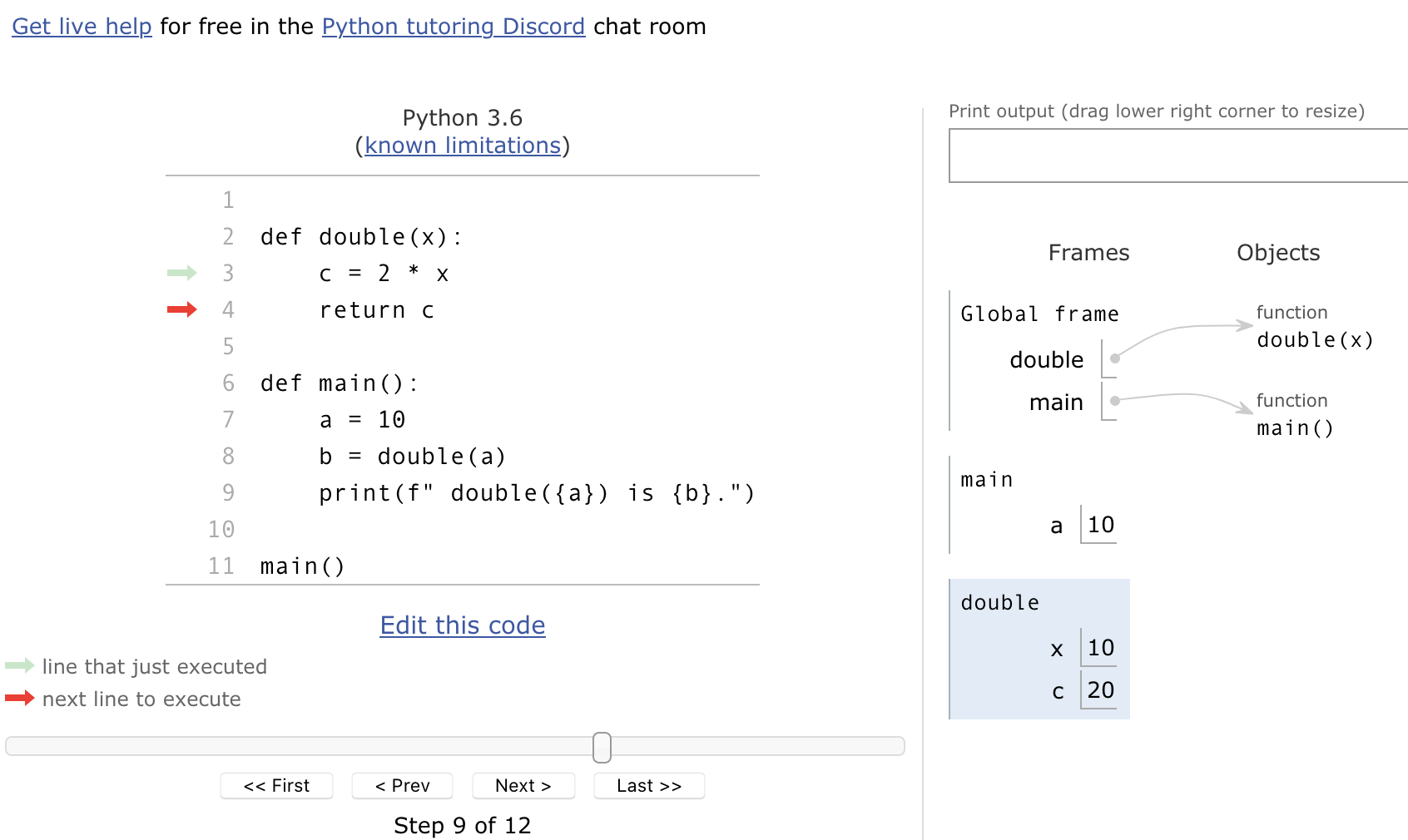# Intro toComputerScience

Fall 2020
##### site-->

# functionsThis is probably the most important material of the term.

Your mission this week is to work on all the different aspects: how to define them, how to run them, when to use them, how to document them, and so on.

Topics to understand :

• What are functions ?
• A mini program within the larger program.
• Has inputs (also called "arguments") and outputs (the "return value")
• Also may have "side effects" : drawing on screen, writing to file, etc
• Define them with the "def name(): ... " syntax
• Invoke (i.e. run) them with the function_name(...) syntax.
• why use them
• splits large tasks into smaller ones
• smaller tasks are easier to understand, document, and test
• avoid code repetition
• variables have "scope" : context in which they are available
• local scope : inside the function when it's running
• global scope : outside the function, before and after it runs
• the specifics of how all that works gets tricky, and varies by language
• input parameters (and similarities / differences with "input()" built-in)
• return value (and similarities / differences with "print()" built-in)
• optional arguments & default values (not done in book - look at an example)
• doc strings (not done in book - look at an example)
• doc tests (optional ; not done in book) - look at an example

I have several videos which will walk you through this stuff.

In looking over what I've written below, I think I have gone too far into the nit-picky weeds several times. Particularly on a first read, skip past anything that isn't making sense. It's the big picture that is important now, particularly if this is your first exposure to these topics.

Do work through these examples yourself on pythontutor.com to see what variables are defined in what context.

## what are functions - the big picture

I told you when this class started that computer science was about managing complexity. Real programs are huge, with thousands of lines of codes and variables. Lots of places that might go wrong.

To make complicated tasks simpler, they are broken into smaller pieces. And often those are pulled apart into even smaller pieces, until the task is small enough to be manageable, one chunk of code that does something specific.

That small piece of code - a function - takes some information, does something, and gives back some other information. Sometimes it also makes a picture, or sends something out over the internet; those are called "side effects". But right now we'll focus on the simplest case, a function with clearly defined inputs (provided to the function before is run) and outputs (what the function produces and sends back).

You can think of a function as a machine :But since python assignment statements like y = f(x) have the input x on the right, and the variable y where the output goes on the left, the machine is better imagined running right to left. Something like this crude representation :

Methods are just one sort of function, ones which have some data already attached - the "object".

## example : the birthday song

Let's look at an example, in several steps.

The first has a function with an input Sally, but no "return". Instead, it just prints to the terminal.

The second returns a string, using python's triple quoted multi-line string syntax. It has a string as input and a different string as output.

The third breaks the task into even smaller pieces: main calls song which calls a different function for each line, with arguments that let them produce slight variations.

That example is pretty silly, but as the tasks get larger and more complicated, the idea of breaking a task into smaller pieces becomes more and more important.

Consider building a large complicated brick building. Each brick needs to be solid and dependable - if the bottom ones crumble, the whole building is in trouble. Similarly, a large complicated program is built upon many small functions, each of which with clear documentation and tests to make sure that it is reliable.

## global and local variables : scope

A function is a "mini program". When it runs, it has its own execution environment and its own variables.

Let's look at an example using pythontutor.com .

Here it is at one step, within the double routine.

There are three different "frames" - execution environments - each with their own variables: the top level program, the main function, and the double function.Here's a trickier example that illustrates some of the issues with global and local variables.

In this first one, a variable name is defined at the top global level.

But then in the function f, it tries to change the value inside that global. In python, doing this creates a ~new~ variable within the function. The global is left unchanged.

In the function g(), it also uses the variable name. But because name hasn't been defined within g(), the global value is used.

# Version 1 : what will be printed ?

name = "Jim"

def f():
name = "Joe"
return 1

def g():
return name

def main():
x = f()
print(" After f(), name is {}".format(name))
print(" From within g(), name is {g()} .")

main()


However, python distinguishes between changing a global itself and changing what's inside a global. This is allowed ... though usually considered not usually good practice, since it can be hard to keep track of what's changing what if you let modifications leak out of functions in places other than the return value.

# Version 2 : what will be printed ?

names = ["Jim", "John", "Mary"]

def f():
names = "Joe"
return 1

def main():
x = f()
print(" names is {}".format(names))

main()


## "pass by value" vs "pass by reference"

Whether the data passed into a program is a copy of the original, or the original itself, varies between programming languages and on the circumstances.

One of the differences between programming languages is how they handle all this.

# Is x modified ?
def double(y):
y = 2*y
return y
x = 10
double_x = double(x)
print("x is ", x)
print("double_x is ", double_x)

# Is numbers modified ?
def double_all(them):
for i in range(len(them)):
them[i] = 2 * them[i]
return them
numbers = [10, 11, 12]
double_numbers = double_all(numbers)
print("numbers is ", numbers)
print("double_numbers is ", double_numbers)


Run these in pythontutor.com to see exactly what's going on ...

## inputs and outputs

A common source of confusion in folks just learning to code is the difference between inputs and outputs to a function, and inputs and outputs to the terminal.

We've been using the built-in function for things like who = input("what is your name?") ; what is typed is input from the terminal.

Similarly, we've been using the built-in function print to send information to the terminal.

Function inputs are those things inside the parens, x and y in foo(x,y).

From within a function, a line like return (a,b,c) statement ends the function, and sends the data (a,b,c). In the calling routine, it's as if the function is replaced by that data.

def plus1(x):
return 1 + x

a = plus1(10)   # the return value replaces plus1(10), leaving "a=11"


You can even send the output from a function back into the same function. This line :

b = plus1(plus1(plus1(10)))


works like a sequence of plus1 machines, with the output from each one getting sent to the input of the next one.

  a  <=  plus1 <= plus1 <=  plus1  <= 10


That's supposed to remind you of the "machine" sketch up above.

To test your understanding, think about what's right and wrong with the the following code :

def f1(x):
print(x)

def f2():
x = input(" ? ")
return x

def f3(x):
return x

y1 = f1(3)                # Oops : f1 has no return value
print(f"y1 is '{y1}'")    # y1 is None , an empty string

y2 = f2()                 # This is fine. y1 is whatever you typed.
print("y2 is '{y2}'"

y3 = f3(20)               # This is fine; y3 is 20
print("y3 is '{}'".format(y3))

print("x is '{}'".format(x))     # Oops : x only exists within the functions, not here.


## keyword arguments : optional with default values

(This is a python-specific feature.)

# An function with optional argument with a default value.

def scale(x, multiplier=2):
""" Return x * multiplier """   #  <= doc string
return multiplier * x

print("twice 10 is {}".format( scale(10) ))
print("triple 10 is {}".format( scale(10, 3) ))


## docstrings

Python has a built-in convention for how code files (also called modules, also called packages, also called libraries ... yes, that can get confusing) and the functions within them should be documented.

The convention is put a triple-quoted string (which is a notation used to specific that the string can span multiple lines) both (a) at the start of the file and (b) right after the first line of a function's definition.

These are are stored as .__doc__ and shown when you ask for help().

For example :

$python >>> def add1(n): ... """ Given a number n, return 1 + n """ ... return 1 + n ... >>> add1(3) 4 >>> add1.__doc__ ' Given a number n, return 1 + n ' >>> help(add1) add1(n) Given a number n, return 1 + n >>>  Here's another example, first without, and then with docstrings : Putting docstrings into your own work is a really good idea - please do so. ## doctests This is optional material for now. Testing is an important part of writing software. Python has various testing methodologies, one of which is called "doctests", which are tests written write into the docstring, both as an example to show how the function is used, and as code that can be verified automatically. doctests look like what would happen at an interactive prompt. If a function double is already defined then you could test it interactively like this. >>> double(10) 20  By adding that to the docstring, and putting some extra code at the bottom of your file, you can automatically check to see if the function behaves in that way. I have an example at doctest_function_example.py $ python doctest_function_example.py -v
Trying:
double(20)
Expecting:
40
ok
Trying:
double('hey')
Expecting:
'heyhey'
ok
__main__
__main__.main
1 items passed all tests:
2 tests in __main__.double
2 tests in 3 items.
2 passed and 0 failed.
Test passed.
What is the value? 10
You said  10 ; double that is  1010

And just for fun ...
nananananananana  batman!



And here's one more example : function_example_3.py

https://cs.bennington.college /courses /fall2020 /introcs /notes /5_functions